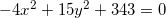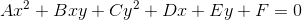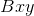# High School Math : Solving Hyperbola Functions

## Example Questions

### Example Question #1 : Pre Calculus

A conic section is represented by the following equation:What type of conic section does this equation represent?

Hyperbola

Ellipse

Parabola

Circle

Hyperbola

Explanation:

The simplest way to know what kind of conic section an equation represents is by checking the coefficients in front of each variable. The equation must be in general form while you do this check. Luckily, this equation is already in general form, so it's easy to see. The general equation for a conic section is the following:Assuming the termis 0 (which it usually is):

• If A equals C, the equation is a circle.
• If A and C have the same sign (but are not equal to each other), the equation is an ellipse.
• If either A or C equals 0, the equation is a parabola.
• If A and C are different signs (i.e. one is negative and one is positive), the equation is a hyperbola.